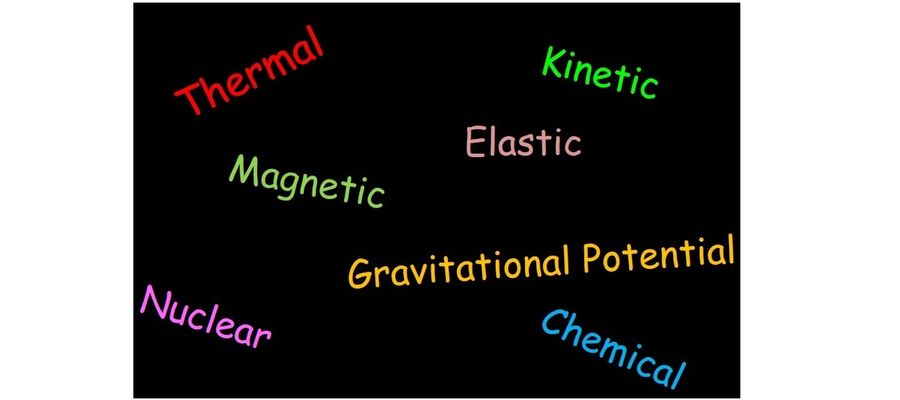# Energy Resources

## You will need a calculator.### Quiz Questions

• 1.
1. An object falls and hits the ground. Which option best describes the energy changes involved?
• A.
Gravitational Potential Energy Store --> Kinetic Energy Store --> Energy transferred to the surroundings as thermal energy store and light waves
• B.
Gravitational Potential Energy Store --> Chemical Energy Store --> Energy transferred to the surroundings as thermal energy store and sound waves
• C.
Gravitational Potential Energy Store --> Kinetic Energy Store --> Energy transferred to the surroundings as thermal energy store and sound waves
• D.
Gravitational Potential Energy Store --> Kinetic Energy Store --> Energy transferred to the surroundings as electrical current in the wires and sound waves
• 2.
2. Which answer below best describes the point(s) where there is the maximum gravitational potential energy?
• A.
A
• B.
B
• C.
C
• D.
A and B
• E.
A and C
• F.
B and C
• 3.
3. Which answer below best describes the point(s) where there is the maximum kinetic energy?
• A.
A
• B.
B
• C.
C
• D.
A and B
• E.
A and C
• F.
B and C
• 4.
4. The pendulum does not swing forever. For it to hypothetically swing forever no friction or air resistance of any kind would be present. This system would be an example of a...?
• A.
A Closed System
• B.
A Vacuum System
• C.
A Frictionless System
• D.
An Energy Neutral System
• 5.
5. Energy cannot be created or destroyed. This statement forms the basis of which principle?
• A.
Pauli Exclusion Principle
• B.
Conservation of energy
• C.
Newtons Third Law
• D.
• 6.
6. A builder pushed a wheelbarrow a distance of 5.0m across flat ground with a force of 50N. How much work was done by the builder?
• A.
10J
• B.
250W
• C.
0.1J
• D.
250J
• 7.
7. When a cyclist brakes, his kinetic energy store is reduced from 1400J to zero in a distance of 7.0m. Calculate the braking force.
• A.
200N
• B.
9800N
• C.
0.005N
• D.
9800J
• 8.
8. A student of weight 450N steps on a box of height 0.2m. Calculate the increase in her gravitational potential energy store.
• A.
2250J
• B.
0.00044N
• C.
90N
• D.
90J
• 9.
9. A weightlifter raises a steel bar of mass 25kg through a height of 1.8m. Calculate the change to the gravitational potential energy store of the bar. Gravitational field strength = 9.8N/kg.
• A.
4.59J
• B.
136.1J
• C.
441J
• D.
1.42J
• 10.
10. Calculate the kinetic energy store of a football of mass 0.44kg moving at a speed of 20m/s.
• A.
45.6J
• B.
8.8J
• C.
4.4J
• D.
88J
• 11.
11. A mobility aid to assist walking uses a steel spring to store energy when the walkers foot goes down, and it returns energy as the foot is lifted. The spring has a spring constant of 250N/m. Calculate the elastic potential energy stored in the spring when its extension is 0.21m.
• A.
26.25J
• B.
5.5J
• C.
52.5J
• D.
11.0J
• 12.
12. An electric motor is used to raise an object. The object's gravitational potential energy store increases by 60J when the motor is supplied with 200J of energy by an electric current. Calculate the percentage efficiency of the motor.
• A.
0.033%
• B.
30%
• C.
3.33%
• D.
333.33%
• 13.
13. Air resistance causes a force on a moving object that opposes its motion. Energy transferred from the object to the surroundings by this force is wasted. How could you improve the efficiency?
• A.
Lubricate the moving parts to reduce friction.
• B.
Cut out noise (e.g. tighten loose parts to reduce vibrations).
• C.
In circuits, use wires with as little electrical resistance as possible.
• D.
Streamline the shapes of moving objects to reduce air resistance.
• 14.
14. A motor transfers 10000J of energy in 25 seconds. Calculate its power.
• A.
0.0025W
• B.
250000W
• C.
250W
• D.
400W
• 15.
15. A 12kW electric shower is used 4 times in a day for 20minutes each time. Calculate the energy supplied to it by electricity in one day.
• A.
960KJ
• B.
57.6KJ
• C.
240000J
• D.
57600KJ

• 1.
Result 1